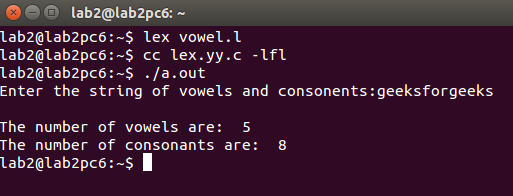Open In App

# LEX program to count the number of vowels and consonants in a given string

Prerequisite: Flex (Fast lexical Analyzer Generator) Given a string containing both vowels and consonants, write a LEX program to count the number of vowels and consonants in given string. Examples:

```Input: Hello everyone
Output: Number of vowels are: 6
Number of consonants are: 7

Input: This is GeeksforGeeks
Output: Number of vowels are: 7
Number of consonants are: 12```

Approach- Approach is very simple. If any vowel is found increase vowel counter, if consonant is found increase consonant counter otherwise do nothing. Below is the implementation:

## C

 `%{``    ``int` `vow_count=0;``    ``int` `const_count =0;``%}` `%%``[aeiouAEIOU] {vow_count++;}``[a-zA-Z] {const_count++;}``%%``int` `yywrap(){}``int` `main()``{``    ``printf``("Enter the string of vowels and consonants:");``    ``yylex();``    ``printf``("Number of vowels are:  %d\n", vow_count);``    ``printf``("Number of consonants are:  %d\n", const_count);``    ``return` `0;``}`

Output: